## Overview

The research activities of our division cover a variety of topics in theoretical and practical aspects of Scientific Computation, which are also pursued in international cooperations. Moreover, numerous lectures and courses are given by the division members in their areas of expertise. The main fields of research are listed in the following:

## Parallel Computation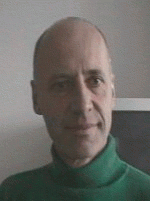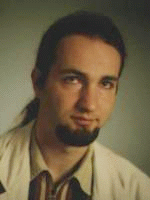• Efficient parallel linear algebra algorithms
• Algorithms for data retrieval in large data collections
• Fast solvers for elliptic partial differential equations
• Signal processing and compression algorithms for media processors

## Mathematical Foundations of Computer Sciences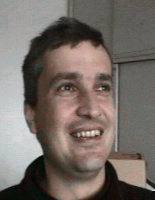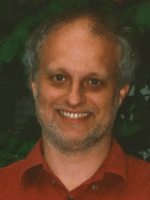• Fourier analysis and wavelet theory
• Numerical integration and approximation of functions in reproducing kernel Hilbert Spaces
• Automatic and semi-automatic deduction
• Algebra of proofs

## Simulation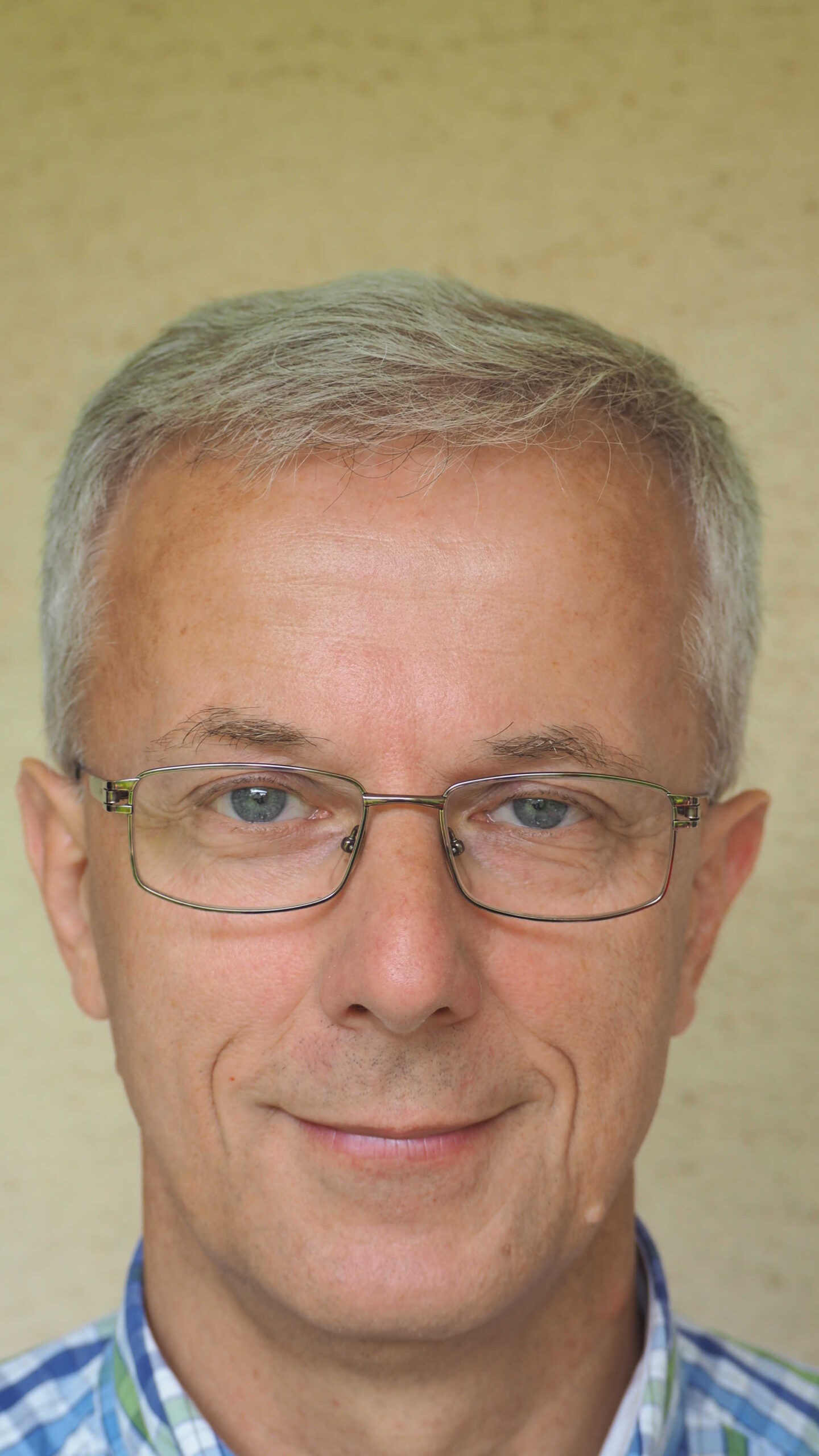• Methods, tools, and applications for discrete event simulation
• Distributed simulation

## Computational Intelligence• Evolutionary artificial neural networks for robots and games
• Simulation of spiking neural networks and other biological systems

Assoc. Univ.-Prof. Dipl.-Ing. Dr. Helmut A. Mayer
Associate Professor